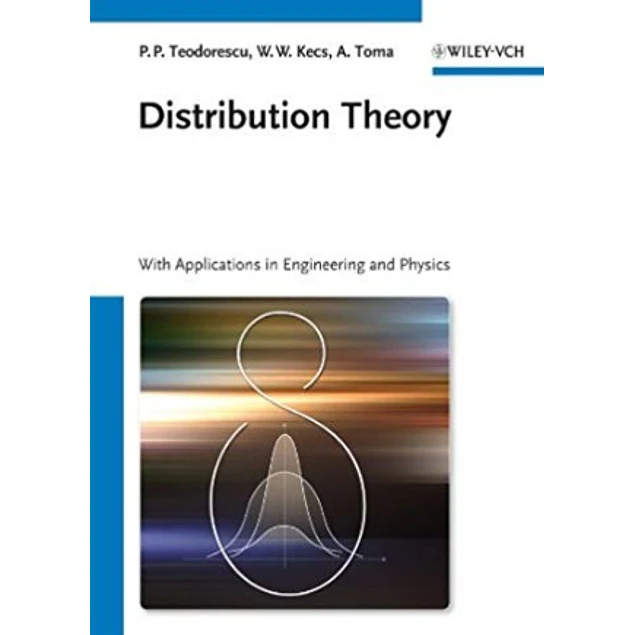## Distribution Theory: With Applications in Engineering and Physics

10.00\$# Distribution Theory: With Applications in Engineering and Physics

10.00\$

1st Edition

by Petre Teodorescu (Author), Wilhelm W. Kecs (Author), Antonela Toma (Author)

In this comprehensive monograph, the authors apply modern mathematical methods to the study of mechanical and physical phenomena or techniques in acoustics, optics, and electrostatics, where classical mathematical tools fail.

They present a general method of approaching problems, pointing out different aspects and difficulties that may occur. With respect to the theory of distributions, only the results and the principle theorems are given as well as some mathematical results. The book also systematically deals with a large number of applications to problems of general Newtonian mechanics, as well as to problems pertaining to the mechanics of deformable solids and physics. Special attention is placed upon the introduction of corresponding mathematical models.

Addressed to a wide circle of readers who use mathematical methods in their work: applied mathematicians, engineers in various branches, as well as physicists, while also benefiting students in various fields.

Year:
2013
Pages:
388
Language:
English
Format:
PDF
Size:
4 MB
ISBN-10:
9.78353E+12
ISBN-13:
978-3527410835
ASIN:
352741083X, B00F0KTCHC
##### Contact
•••••